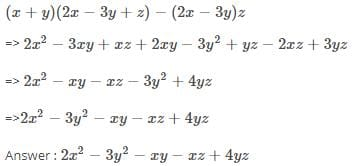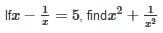Olympiad Test: Algebraic Expressions And Identities

# Olympiad Test: Algebraic Expressions And Identities

Test Description

## 20 Questions MCQ Test Mathematics (Maths) Class 8 | Olympiad Test: Algebraic Expressions And Identities

Olympiad Test: Algebraic Expressions And Identities for Class 8 2023 is part of Mathematics (Maths) Class 8 preparation. The Olympiad Test: Algebraic Expressions And Identities questions and answers have been prepared according to the Class 8 exam syllabus.The Olympiad Test: Algebraic Expressions And Identities MCQs are made for Class 8 2023 Exam. Find important definitions, questions, notes, meanings, examples, exercises, MCQs and online tests for Olympiad Test: Algebraic Expressions And Identities below.
Solutions of Olympiad Test: Algebraic Expressions And Identities questions in English are available as part of our Mathematics (Maths) Class 8 for Class 8 & Olympiad Test: Algebraic Expressions And Identities solutions in Hindi for Mathematics (Maths) Class 8 course. Download more important topics, notes, lectures and mock test series for Class 8 Exam by signing up for free. Attempt Olympiad Test: Algebraic Expressions And Identities | 20 questions in 20 minutes | Mock test for Class 8 preparation | Free important questions MCQ to study Mathematics (Maths) Class 8 for Class 8 Exam | Download free PDF with solutions
 1 Crore+ students have signed up on EduRev. Have you?
Olympiad Test: Algebraic Expressions And Identities - Question 1

### Which of the following is an expression?

Detailed Solution for Olympiad Test: Algebraic Expressions And Identities - Question 1 (c) Becoz highest power of variable 'x' is not equal to zero . ..
Olympiad Test: Algebraic Expressions And Identities - Question 2

### Which of the following is a binomial?

Detailed Solution for Olympiad Test: Algebraic Expressions And Identities - Question 2

An algebraic expression consisting of unlike terms is called a binomial.

so, 2x+7 is a binomial as it contains two unlike terms i.e 2x and 7.

Olympiad Test: Algebraic Expressions And Identities - Question 3

### Expressions consists of _____________ and _______________.

Detailed Solution for Olympiad Test: Algebraic Expressions And Identities - Question 3 Variables and constants .This is because an expression is made up of these only.
Olympiad Test: Algebraic Expressions And Identities - Question 4

What degree does x3 - x2y2 - 8y2+ 2 have?

Detailed Solution for Olympiad Test: Algebraic Expressions And Identities - Question 4

Monomial means expression containing only one term and option B has one term so it is a monomial.

Olympiad Test: Algebraic Expressions And Identities - Question 5

When numbers/literals are added or subtracted, they are called _________.

Olympiad Test: Algebraic Expressions And Identities - Question 6

Multiply (a + 7) and (b – 5).

Detailed Solution for Olympiad Test: Algebraic Expressions And Identities - Question 6

(a+7)(b-5) = a(b-5)+7(b-5) = ab-5a+7b-35

Olympiad Test: Algebraic Expressions And Identities - Question 7

Simplify: (x + y) (2x – 3y + z) – (2x – 3y)z

Detailed Solution for Olympiad Test: Algebraic Expressions And Identities - Question 7Olympiad Test: Algebraic Expressions And Identities - Question 8

If (x+1/x) = 6, find  x2+1/x2

Detailed Solution for Olympiad Test: Algebraic Expressions And Identities - Question 8 X²+1/x² can be written as (x+1/x)²-2 on putting values we have 6²-2=34
Olympiad Test: Algebraic Expressions And Identities - Question 9Olympiad Test: Algebraic Expressions And Identities - Question 10

Which of the following is an expression?

Detailed Solution for Olympiad Test: Algebraic Expressions And Identities - Question 10 Option C is correct bcuz, an expression consists of at least one variable like :- x, y, z as well as a number. An expression can also be like this :- 2xy, 3y², 5xy+8, 6z²-3, etc
Olympiad Test: Algebraic Expressions And Identities - Question 11

Which of the following is a trinomial?

Detailed Solution for Olympiad Test: Algebraic Expressions And Identities - Question 11 Because monomial 1 term binomial, 2 terms and trinomial 3 terms therefor 7_3x+4y trinomial.
Olympiad Test: Algebraic Expressions And Identities - Question 12

Which of the following is like term as 3xy2?

Olympiad Test: Algebraic Expressions And Identities - Question 13

Subtract 3x (x – 4y + 5z) from 4x (2x – 3y + 10z).

Olympiad Test: Algebraic Expressions And Identities - Question 14

n (4 + m) = 4n + ___

Detailed Solution for Olympiad Test: Algebraic Expressions And Identities - Question 14 N(4+m) = 4n + ___Here , n is multiplied by 4 which is added to n multiplied by m.so, n×4= 4n n×m= nm so the answer is 4n + nm.
Olympiad Test: Algebraic Expressions And Identities - Question 15

Using identity evaluate 297 × 303.

Detailed Solution for Olympiad Test: Algebraic Expressions And Identities - Question 15
297×303
(300-3)(300+3)
(300)whole square (3)whole square
90000-9
89991. identity
{a-b} {a+b} ={a square} - {b square}
Olympiad Test: Algebraic Expressions And Identities - Question 16

Simplify: (1.5x – 4y)(1.5x + 4y +3) – 4.5x +12y

Detailed Solution for Olympiad Test: Algebraic Expressions And Identities - Question 16
(1.5x - 4y) (1.5x + 4y + 3) - 4.5x + 12y
= 1.5x(1.5x + 4y +3) - 4y(1.5x + 4y +3) - 4.5x +12y
= (1.5x X 1.5x) + (1.5x X 4y) + (1.5x X 3) - (4y X 1.5x) - (4y X 4y) - (4y x 3) - 4.5x +12y

= 2.25x^2 + 6xy + 4.5x - 6xy - 16y^2 - 12y - 4.5x + 12y
= 2.25x^2 + ( 6 - 6)xy + (4.5 - 4.5)x - 16y^2 + (12 - 12)y
= 2.25x^2  + 0 + 0 - 16y^2 + 0
= 2.25x^2 - 16y^2
Olympiad Test: Algebraic Expressions And Identities - Question 17

If x + y = 12 and xy = 32, find the value of x2+ y2.

Detailed Solution for Olympiad Test: Algebraic Expressions And Identities - Question 17
x=8 or 4 and y=4 or 8 so,x^2+y^2=64 +16 or 16 +64 =80 and that is option A.
Olympiad Test: Algebraic Expressions And Identities - Question 18

(x – a) (x + a) = ?

Detailed Solution for Olympiad Test: Algebraic Expressions And Identities - Question 18 (a-b)(a+b) = a^2 - b^2(x-a)(x+a) = x (x+a) -a (x+a) = x^2 + ax -ax -a^2 = x^2 - a ^2
Olympiad Test: Algebraic Expressions And Identities - Question 19

Which of the following is a trinomial?

Olympiad Test: Algebraic Expressions And Identities - Question 20

If x = 3 is solution of x² + kx + 15, value of k is

Detailed Solution for Olympiad Test: Algebraic Expressions And Identities - Question 20

Since solution is x=3, this means the equation is equal to 0 when x=3, substituting the values gives k=-8

## Mathematics (Maths) Class 8

197 videos|360 docs|48 tests(Scan QR code)